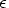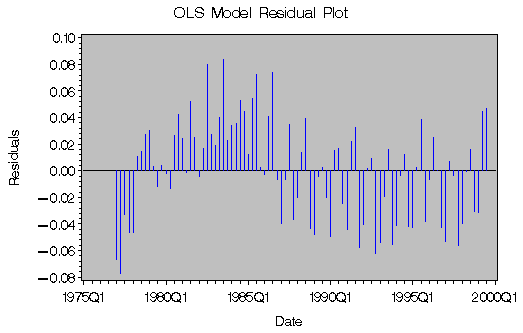# SAS/ETS Examples

## Calculating Price Elasticity of Demand

Contents | SAS Program

# OverviewFigure 1: Quantity and Price for Beef

The price elasticity of demand is defined as the percentage change in quantity demanded for some good with respect to a one percent change in the price of the good. For example, if the price of some good goes up by 1%, and as a result sales fall by 1.5%, the price elasticity of demand for this good is -1.5%/1% = -1.5. Thus, price elasticity measures responsiveness of quantity demanded to changes in price. Price elasticity greater than one is called price elastic, and price elasticity less than one is called price inelastic. A given percentage increase in the price of an elastic good will reduce the quantity demanded for the good by a higher percentage than for an inelastic good. In general, a necessary good is less elastic than a luxury good. For an introductory text on price elasticities, see Nicholson (1992). Price elasticity can be expressed as:whereis the price elasticity, P is the price of the good, and Q is the quantity demanded for the good.

# Analysis

In this example, you will calculate the price elasticity of demand for beef in a simple log-linear demand model. The data consist of quarterly retail prices and per capita consumption for beef. The data period covers the first quarter of 1977 through the third quarter of 1999. The data were obtained from the USDA Red Meats Yearbook (accessed 2001).

The log-linear demand model is of the following form:

lnQ = a + b·lnP

where Q and P are defined as before, a and b are parameters to be estimated.

The log-linear demand model is a very simple one. In the real world, there may be many additional complexities that need to be considered in the model. For example, prices of some other closely related goods may have a significant effect on the quantity demanded for beef; hence, they may also enter the right-hand side of the demand equation. On a store level, there may also be occasions when sales remain zero regardless of how much the price is; for example, when the good is out of stock. For demonstration purposes these complexities will be ignored.

The log-linear demand function implies that the price elasticity of demand is constant:Thus, to obtain an estimate of the price elasticity, you just need an estimate of b. You can use the AUTOREG procedure to obtain the estimates.

To use the AUTOREG procedure, you first read in the price and quantity data in the DATA step, and transform the price and quantity data into log forms:

      data a ;
input yr qtr q p ;
date = yyq(yr,qtr) ;
format date yyq6. ;
lq = log(q) ;
lp = log(p) ;
datalines;
1977   1   22.9976   142.1667
1977   2   22.6131   143.9333
1977   3   23.4054   146.5
1977   4   22.7401   150.8
1978   1   22.0441   160

... more datalines ...

1997   4   16.2354   279.3
1998   1   16.6884   273.4667
1998   2   17.1985   278.1
1998   3   17.5085   277.3667
1998   4   16.6475   279.5333
1999   1   16.6785   278
1999   2   17.7635   284.7667
1999   3   17.6689   289.2333
;
run ;


Then you specify the input data set in the PROC AUTOREG statement and specify the regression model in a MODEL statement. If you are not concerned with autocorrelated errors and just want to do an ordinary least-squares regression, you can specify the model as follows:

    proc autoreg data=a outest=estb ;
model lq = lp ;
output out=out1 r=resid1 ;
title "OLS Estimates";
run ;


This will yield ordinary least squares estimates of a and b. The output is shown in Figure 2.

 OLS Estimates

The AUTOREG Procedure

Ordinary Least Squares Estimates
SSE 0.12252634 DFE 89
MSE 0.00138 Root MSE 0.03710
SBC -334.26774 AIC -339.28946
Regress R-Square 0.8521 Total R-Square 0.8521
Durbin-Watson 1.1073

Variable DF Estimate Standard Error t Value Approx
Pr > |t|
Intercept 1 5.8364 0.1294 45.10 <.0001
lp 1 -0.5314 0.0235 -22.64 <.0001

Figure 2: OLS Esimates

The parameter estimate for b is equal to -0.5314, which suggests that increasing the price for beef by 1% will reduce the demand for beef by 0.53%.

The following SAS code uses the GPLOT procedure to plot the residuals obtained from the OLS estimation, as shown in Figure 3.

    proc gplot data=plot2 ;
title 'OLS Model Residual Plot' ;
axis1 label=(angle=90 'Residuals') ;
axis2 label=('Date') ;
symbol1 c=blue i=needle v=none ;
plot resid1*date / cframe=ligr haxis=axis2 vaxis=axis1 ;
run ;Figure 3: OLS Model Residual Plot

Note that the residual errors from the OLS regression do not appear to be random. The residual plot shows evidence of autocorrelation in the error process. This violates the independent error assumption of the classical regression model, and the OLS estimates will be inefficient. Thus, the parameter estimates are not as accurate as they could be.

To improve the efficiency of the estimated parameters, you can correct for autocorrelation using the AUTOREG procedure. Specify the order of autocorrelation using the NLAG= option. Since the data are quarterly, this example chooses order 1 as well as order 4 for seasonality considerations. You can use the METHOD= option to specify the maximum likelihood estimation.

   proc autoreg data=a outest=estb ;
model lq = lp  / nlag=(1 4) method=ml ;
output out=out2 r=resid2 ;
title "OLS, Autocorrelations, & Maximum Likelihood Estimates";
run ;


The output in Figure 4 first shows the initial OLS results. Then the estimates of autocorrelations and the maximum likelihood estimates are displayed.

 OLS, Autocorrelations, & Maximum Likelihood Estimates

The AUTOREG Procedure

Ordinary Least Squares Estimates
SSE 0.12252634 DFE 89
MSE 0.00138 Root MSE 0.03710
SBC -334.26774 AIC -339.28946
Regress R-Square 0.8521 Total R-Square 0.8521
Durbin-Watson 1.1073

Variable DF Estimate Standard Error t Value Approx
Pr > |t|
Intercept 1 5.8364 0.1294 45.10 <.0001
lp 1 -0.5314 0.0235 -22.64 <.0001

Estimates of Autocorrelations
Lag Covariance Correlation -1 9 8 7 6 5 4 3 2 1 0 1 2 3 4 5 6 7 8 9 1
0 0.00135 1.000000 |                    |********************|
1 0.000564 0.419098 |                    |********            |
2 -0.00001 -0.007458 |                    |                    |
3 0.000459 0.340944 |                    |*******             |
4 0.000895 0.664708 |                    |*************       |

 OLS, Autocorrelations, & Maximum Likelihood Estimates

The AUTOREG Procedure

Maximum Likelihood Estimates
SSE 0.04786518 DFE 87
MSE 0.0005502 Root MSE 0.02346
SBC -407.51126 AIC -417.55469
Regress R-Square 0.6109 Total R-Square 0.9422
Durbin-Watson 1.4299

Variable DF Estimate Standard Error t Value Approx
Pr > |t|
Intercept 1 5.1843 0.1929 26.87 <.0001
lp 1 -0.4131 0.0353 -11.69 <.0001
AR1 1 -0.1765 0.0713 -2.48 0.0152
AR4 1 -0.7134 0.0723 -9.87 <.0001

Figure 4: OLS,Autocorrelation,and Maximum Likelihood Estimates

By including an autoregressive error structure in the model, the total R2 statistic increases from 0.8521 to 0.9422 in the maximum likelihood estimation. The information criteria AIC and SBC become more negative. These imply significant improvement over the OLS estimation.

Notice also that the estimate for b changes from -0.5314 to -0.4131, which means that the estimated elasticity for beef demand is smaller in absolute value than the OLS estimate. Since the estimated elasticity is smaller than 1 in absolute value, you may conclude that demand for beef is relatively inelastic.

Figure 5 illustrates the residuals obtained from the autoregressive error model estimation.

    proc gplot data=plot2 ;
title 'Autoregressive Error Model Residual Plot' ;
axis1 label=(angle=90 'Residuals') ;
axis2 label=('Date') ;
symbol1 c=blue i=needle v=none ;
plot resid2*date / cframe=ligr haxis=axis2 vaxis=axis1 ;
run ;Figure 5: Autoregressive Error Model Residual Plot

# References

Nicholson, W. (1992), Microeconomic Theory: Basic Principles and Extensions, Fifth Edition, Fort Worth: Dryden Press.

SAS Institute Inc. (1999), SAS/ETS User's Guide, Version 8, Cary, NC: SAS Institute Inc.

USDA-ERS Electronic Data Archive, Red Meats Yearbook, housed at Cornell University's Mann Library, [http://usda.mannlib.cornell.edu/], accessed 25 September 2001.